output: rmarkdown::html_vignette author: “Warren Porter & Michael Kearney” vignette: > % % % editor_options: chunk_output_type: console —

## 1. Overview

This document shows the derivation of equations for time-dependent temperature change in objects and organisms, drawing from and building on the equations and ideas presented in Porter et al. 1973. It considers the issue of whether to model the system as ‘one lump’ or ‘two lumps’. It develops an analytical solution for the one lump scenario under constant conditions where metabolism can be neglected. It also derives the equations for including metabolic heat generation. Equations suitable for use with an ordinary differential equation (ODE) solver are provided for the one and two lump scenarios. Implementations of these equations are found in the ‘onelump’, ‘onelump_var’ and ‘twolump’ functions of NicheMapR.

## 2. Symbols used

Symbols Definition Units
$$a$$ constant -
$$A$$ area $$m^2$$
$$b$$ constant -
$$C$$ thermal capacitance, $$mc_p$$ kg-J/(kg-°C)
$$C_1$$,$$C_2$$ constants none
$$c_p$$ specific heat J/kg-°C
$$E$$ voltage volts
$$F$$ configuration factor -
$$h$$ heat transfer coefficient W/(m$$^2$$-°C)
$$I$$ current amps
$$j$$ constant C
$$k$$ thermal conductivity W/(m-°C)
$$L$$ characteristic dimension m
$$m$$ mass kg
$$\dot{m}$$ mass flow rate kg/s
$$P_1$$,$$P_2$$ constants none
$$P$$ d/dt place holder to ease algebraic operations
$$q^{'''}$$ volume-specific heat generation W/m$$^3$$
$$Q$$ heat flux W
$$R$$ thermal resistance 1/(W-C)
$$S^2$$ ellipsoid geometric fraction m$$^2$$
$$t$$ time s
$$T$$ temperature °C
$$v$$ wind speed m/s
$$V$$ volume m$$^3$$
$$x$$ length m
$$\epsilon$$ emissivity -
$$\mu$$ dynamic viscosity kg/(s-m)
$$\rho$$ density kg/m$$^3$$
$$\sigma$$ Stefan-Boltzmann constant W/(m$$^2$$-K$$^4$$)
$$\tau$$ time constant s
$$\theta$$ inverse of $$\tau$$ 1/s
Subscripts Definition
$$a$$ air
$$b$$ body
$$bl$$ blood
$$c$$ core
$$conv$$ convection
$$e$$ environment
$$evap$$ evaporation
$$f$$ final
$$gen$$ generated
$$i$$ initial
$$met$$ metabolism
$$o$$ object
$$rad$$ radiation
$$s$$ surface
$$sk$$ skin
$$sol$$ solar
$$st$$ stored

## 3. General system diagram and Ohm’s Law analogy

We assume the animal in cross section has a region of uniform temperature in the core, $$T_c$$, and a shell of lower (or higher) temperature with a measurable skin temperature, $$T_{sk}$$, and a measurable skin surface temperature, $$T_s$$. We then obtain the system diagram in Figure 1.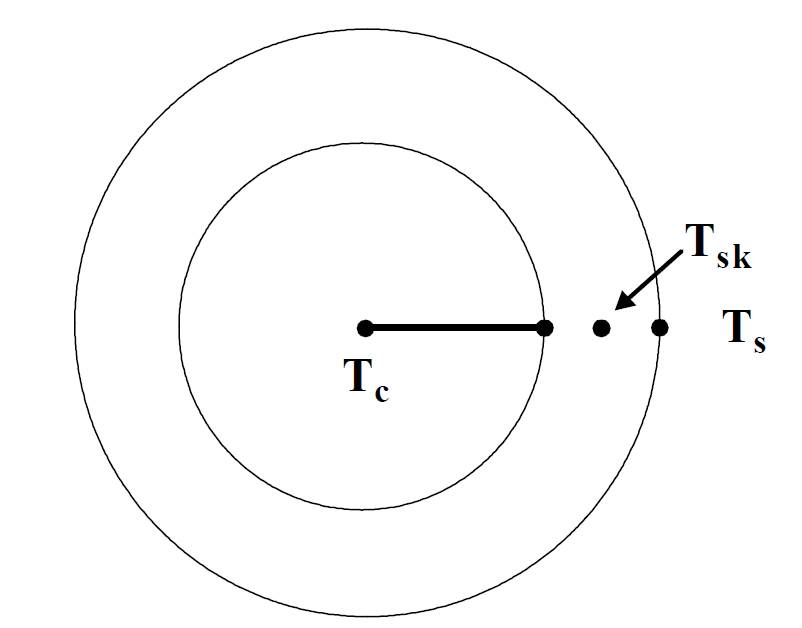A cross section system diagram for heat flow in an animal

We use an Ohm’s Law analogy for heat flow, where temperature gradients are treated as voltages, heat flow as current flow and properties affecting thermal resistance to heat flow (area, shape, thermal conductivity) as electrical resistance. The thermal circuit for the system in Figure 1, describing all possible heat flows in the cross section, is shown in Figure 2.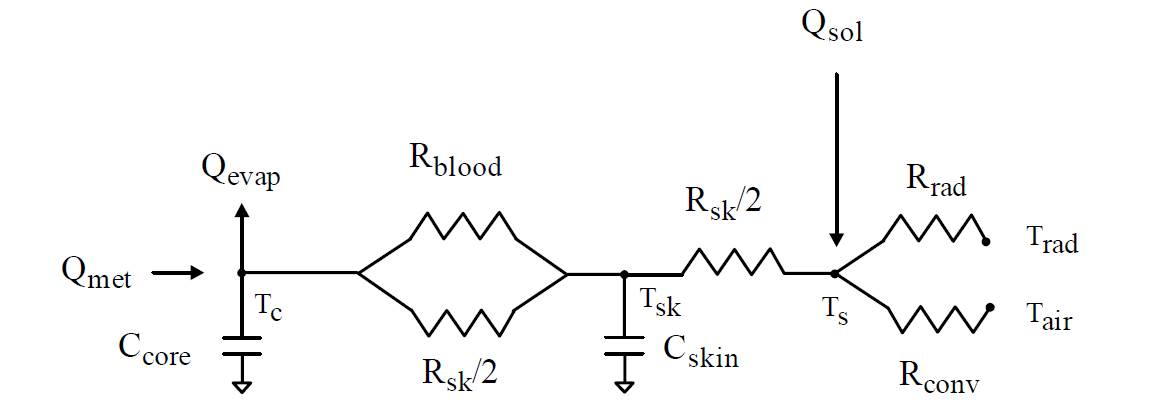An equivalent thermal circuit diagram for the system diagram in Figure 1. This assumes a point source of heat at the centre and a slab approximation of the geometry

The thermal resistances are:

$\begin{equation} R_{sk} = \frac{x_{sk}}{k_{sk}A} \end{equation}$

$\begin{equation} R_{bl} = \frac{1}{\dot{m}_{bl} c_p} \end{equation}$

$\begin{equation} R_{conv} = \frac{1}{h_{conv}A} \end{equation}$

$\begin{equation} R_{rad} = \frac{T_s-T_{rad}}{\epsilon \sigma F_{o-e} A(T_s^4-T_{rad}^4)} \end{equation}$

## 4. One lump or two? Computing internal to external resistance ratios

As discussed in detail in Porter et al. 1973, if internal resistances can be shown to be small (< 10%), relative to the external resistances, the animal can be assumed to be uniform in temperature and can be simulated as ‘one lump’, where only core and outer surface temperature are solved for. Otherwise, one requires a ‘two lump’ model that simultaneously solves for core, skin temperature and outer surface temperature.

To make this judgment, the separate components of internal and external resistances must be condensed into equivalent resistances. How this is done depends on whether the resistances are in series or parallel. In Fig. 2 the blood and flesh resistances between the core and skin nodes are in parallel, while they together are in series with the skin resistance between the skin and outer surface nodes.

According to Ohm’s law, resistance $$R$$ is the ‘voltage’ $$E$$ (in our analogy, the temperature difference) divided by the ‘current’ $$I$$ (heat flow), $$R = E/I$$.

When two resistances $$R_1$$ and $$R_2$$ are in series, the current passess through both thus

$\begin{equation} E = IR_1 + IR_2 = I(R_1 + R_2) = IR_T \end{equation}$

where

$\begin{equation} R_T = R_1 + R_2. \end{equation}$

When the resistances are parallel, the voltage is the same across both $$R_1$$ and $$R_2$$ and the total current flow must be partitioned among the two paths. Thus, $$I_T = I_1 + I_2$$. But by Ohm’s law $$I_1 = E/R_1$$ and $$I_2 = E/R_2$$, so $$I_T = E/R_1 + E/R_2 = E/R_T$$. Dividing through by $$E$$ and rearranging we have

$\begin{equation} R_T = \frac{R_1 R_2}{R_1 + R_2}. \end{equation}$

We can use these ideas to determine the relative magnitude of the external resistance to heat flow vs. the internal resistance to heat flow - this ratio is called the Biot number. Porter et al. 1973 obtained the following parameters for heat flow in a 40 g Desert Iguana $$Dipsosaurus dorsalis$$ that can be used to define resistances (using a blood flow rate based on a cardiac output of 3.5 g min$$^{-1}$$ 100 $$g^{-1}$$):

$$c_p$$ = 0.83 cal g$$^{-1}$$ °C$$^{-1}$$ = 3474 J kg$$^{-1}$$ °C$$^{-1}$$

$$A$$ = 140 cm$$^2$$ = 0.014 m$$^2$$

$$x_{sk}$$ = 0.1 cm = 0.001 m

$$k_{sk}$$ = 0.072 cal cm$$^{-1}$$ min$$^{-1}$$ °C$$^{-1}$$ = 0.502 $$W$$ m$$^{-1}$$ °C$$^{-1}$$

$$\dot{m}_{bl}$$ = 3.5 g min$$^{-1}$$ 100 $$g^{-1}$$ = 1.4 g min$$^{-1}$$ = 2.33e-05 kg s$$^{-1}$$

$$h_{conv}$$ (wind speed 2.1 to 0.45 m s$$^{-1}$$) = 0.036 to 0.012 cal cm$$^{-2}$$ min$$^{-1}$$ °C$$^{-1}$$ = 8.37-25.12 W m$$^{-2}$$ °C$$^{-1}$$.

Thus, from eqs. 1-4, respectively, we get

$$R_{sk} = \frac{0.001}{2 \cdot 0.502 \cdot 0.014}$$ = 0.071 $$^{\circ} C$$ W$$^{-1}$$

$$R_{bl} = \frac{1}{2.33e-05 \cdot 3474}$$ = 12.34 $$^{\circ} C$$ W$$^{-1}$$

$$R_{conv} = \frac{1}{(25.12 \: to \: 8.37) \cdot 0.014}$$ = 2.84 to 8.53 $$^{\circ} C$$ W$$^{-1}$$

$$R_{rad} = \frac{T_s-297}{1 \cdot 5.67 \cdot 10^{-8} \cdot 0.8 \cdot 0.014 \cdot (T_s^4-297^4)}$$ = 14.07 to 14.87 $$^{\circ} C$$ W$$^{-1}$$

assuming a surface temperature from 26 °C to 37 °C and a radiant temperature of 24 °C.

Using eq. 7, the total resistance in the body is

$$R_b = \frac{R_{sk} R_{bl}}{R_{sk} + R_{bl}} = \frac{0.071 \cdot 12.34}{0.071 + 12.34} =$$ 0.071

and that of the environment is

$$R_e = \frac{R_{conv} R_{rad}}{R_{conv} + R_{rad}} = \frac{2.84 \cdot 14.07}{2.84 + 14.07} =$$ 2.36

and $$R_b/R_e$$ = 0.006. Since $$R_e$$ is over 33 times greater than $$R_b$$, $$R_e$$ is controlling heat loss and a 40 g lizard like the desert iguana may be treated as a single lump.

Figure 3 shows calculations of Biot numbers for a range of body sizes.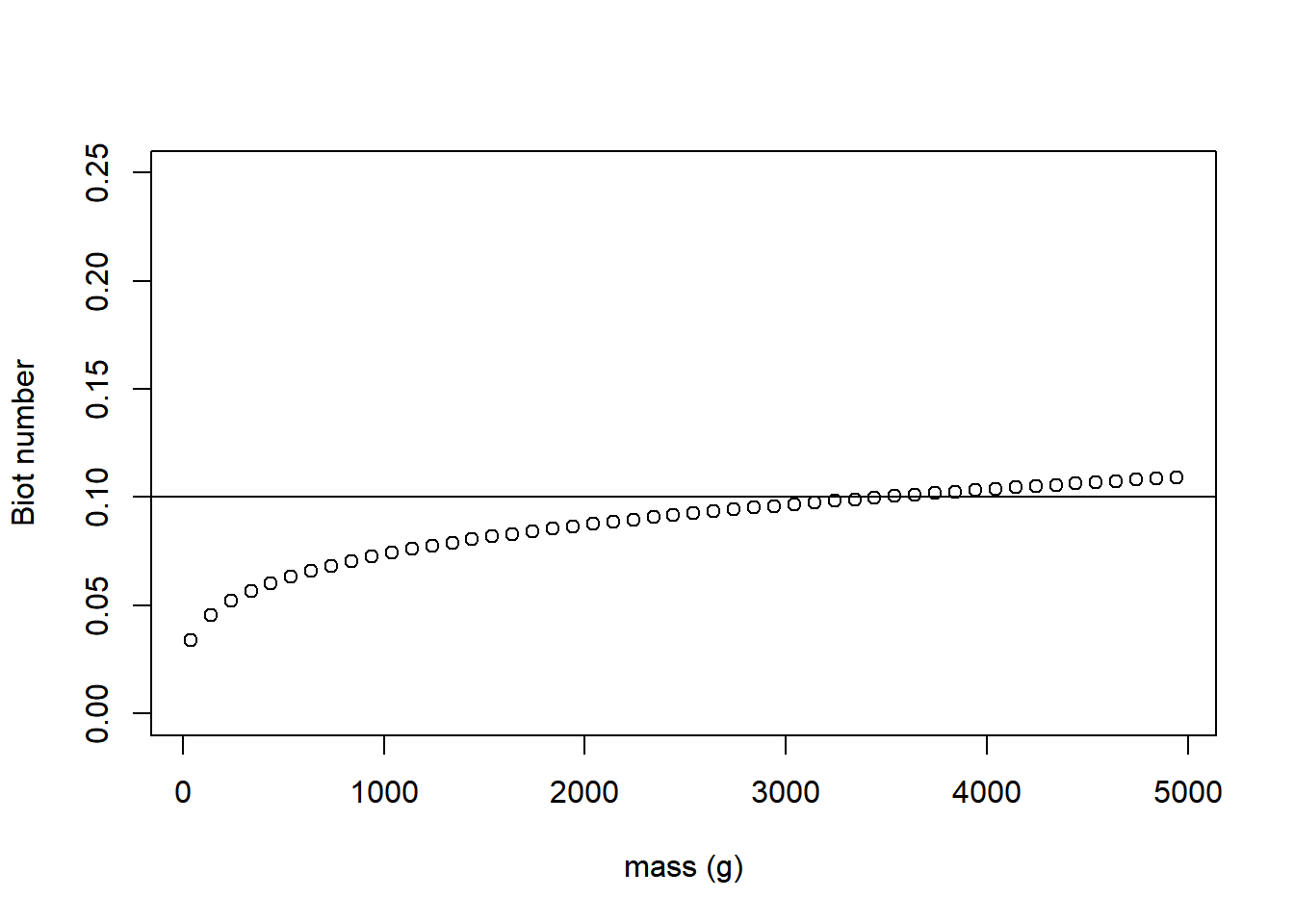The ratio of internal and external resistance (Biot number) for desert iguana-shaped lizards of different body mass at 0.45 m/s wind speed, 24 °C air and radiant temperature, 26 °C skin temperature, and flesh thermal conductivity of 0.5 W/mC.

## 5. Derivation of the one-lump solution

When the Biot number is sufficiently low (< 0.1), uniform body temperature can be assumed and the system diagram of Fig. 2 reduces to Fig. 4.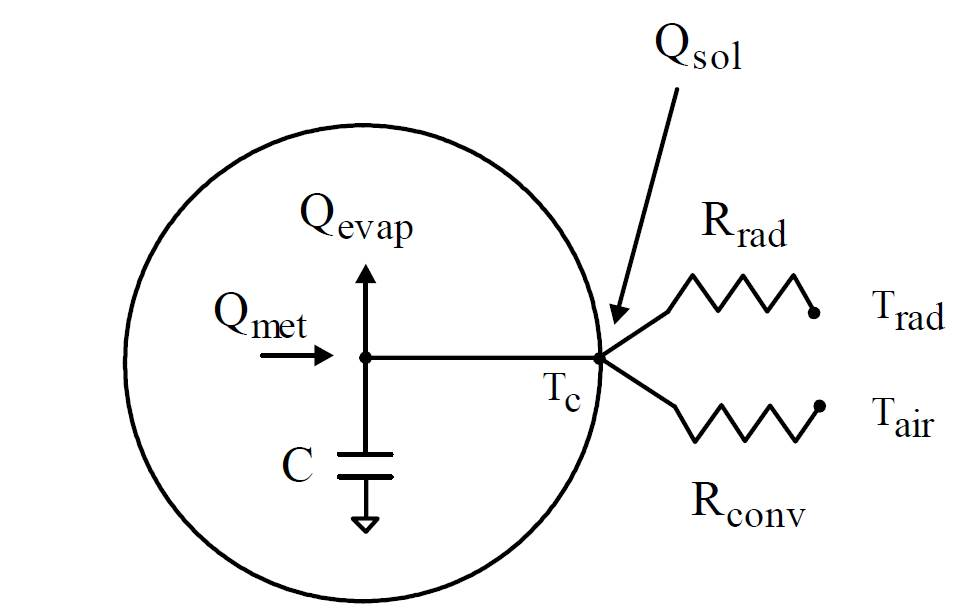A one-lump model of heat loss assuming a point (line) source of heat in the centre of the cylindrical geometry

The thermal capacitance, $$C$$, is the product of mass and specific heat, $$mc_p$$. Notice here that the energy input due to metabolism is assumed to be generated at the core ‘node’ and is connected by a ‘short circuit’ to the skin node. Using the system diagram to write the heat balance equation $$Q_{in} + Q_{gen} = Q_{out} + Q_{st}$$ or, more specifically,

$\begin{equation} Q_{sol} + Q_{met} = Q_{evap} + \frac{T_c - T_{a}}{R_{conv}} + \frac{T_c - T_{rad}}{R_{rad}} + C \frac{dT_c}{dt}. \end{equation}$

Collecting all the temperatures on the left and assuming core temperature-independent constants on the right

$\begin{equation} \frac{T_c - T_{a}}{R_{conv}} + \frac{T_c - T_{rad}}{R_{rad}} + C \frac{dT_c}{dt} = Q_{sol} + Q_{met} - Q_{evap} \end{equation}$

$\begin{equation} C \frac{dT_c}{dt} + \frac{T_c}{R_{conv}} + \frac{T_c}{R_{rad}} = Q_{sol} + Q_{met} - Q_{evap} + \frac{T_{a}}{R_{conv}} + \frac{T_{rad}}{R_{rad}} \end{equation}$

and multiplying all terms by $$R_{conv} \cdot R_{rad}$$ to clear the denominators:

$\begin{equation} C R_{conv} R_{rad} \frac{dT_c}{dt} + T_c R_{rad} + T_c R_{conv} = Q_{sol} R_{conv} R_{rad} + (Q_{met} - Q_{evap}) R_{conv} R_{rad} + T_{a} R_{rad} + T_{rad} R_{conv}. \end{equation}$

Porter et al. 1973 have shown that $$Q_{met} - Q_{evap} \approx 0$$ over the temperature range of activity of the desert iguana. Therefore we can neglect those terms. We put the terms assumed to be independent of core temperature on the right and divide throughout by the coefficient of the derivative to obtain a general form for solution of an ordinary homogeneous differential equation:

$\begin{equation} \frac{dT_c}{dt} + [\frac{R_{rad} + R_{conv}}{C R_{conv} R_{rad}}] T_c = \frac{Q_{sol}}{C} + \frac{T_{a}}{C R_{conv}} + \frac{T_{rad}}{C R_{rad}}. \end{equation}$

### Solving the differential equation

Equation 12 is of the form

$\begin{equation} \frac{dy}{dt} + \theta y = j \end{equation}$

Equation 13 is a homogenous ordinary differential equation where $$\theta$$ and $$j$$ are here assumed to be constants to be determined. The mechanism of the solution is

$\begin{equation} y = y_p + y_c \end{equation}$

to solve the steady state part, $$y_p$$, also known as the particular integral, and the transient part, $$y_c$$, also known as the complimentary function. Here $$y$$ represents the core temperature, $$T_c$$.

### The complimentary function

To solve $$y_c$$ let $$j$$ in 13 = 0. Thus, 13 is

$\begin{equation} \frac{dy}{dt} + \theta y = 0 \end{equation}$

For convenience we can define an operator $$P = \frac{d}{dt}$$, to solve in an algebraic form rather than a calculus format:

$\begin{equation} Py = \frac{dy}{dt}. \end{equation}$

We can substitue 13 into 14 to obtain

$\begin{equation} Py + \theta y = 0 \end{equation}$

The solution to 17 is trivial if $$y = 0$$, so we will assume that it is $$p + \theta = 0$$. Thus

$\begin{equation} y(P + \theta) = 0 \end{equation}$

and

$\begin{equation} P = -\theta. \end{equation}$

The solution of a homogeneous first order ordinary linear differential equation, such as 15, is always of the form

$\begin{equation} y_c = C_1 e^{pt}, \end{equation}$

as discussed below.

Substituting 19 in to 20

$\begin{equation} y_c = C_1 e^{-\theta t}. \end{equation}$

The integration constant, $$C_1$$, is never evaluated until the entire equation, 13, is solved. The reader can verify that 21 is the correct solution by rearranging 15 such that $$\frac{dy}{y} = -\theta dt$$, integrating directly and taking antilogs to obtain 21. The $$P$$ operator becomes more useful as the problems get more complex, as in the two lump solution below.

### The particular integral

The particular integral, $$y_p$$, is the steady state condition at the end of the transient, i.e. the ‘operative environmental temperature’ (Winslow et al., 1940; Bakken et al. 1985) in our case. This is represented by $$j$$, the ‘forcing function’, in equation 13.

If we assume that $$y_p = A$$, a constant, then $$dy_p / dt = 0$$ at steady state. Thus, for the particular integral, equation 13 becomes

$\begin{equation} 0 + \theta y_p = A = j \end{equation}$

so

$\begin{equation} y_p = \frac{j}{\theta}. \end{equation}$

The solution is the sum of the particular integral (steady part), and the complimentary function (transient part), as stated in equation 14.

We have from 19 and 20

$\begin{equation} y = T_c = C_1e^{-\theta t} + \frac{j}{\theta}. \end{equation}$

Now that we have the full solution, we can evaluate the integration constant, $$C_1$$. Since the independent variable is time, not distance, one time dependent condition might be the initial condition at $$t = 0$$. At that time the core temperature is the inital (starting) core temprature of the transient, $$T_c = T_{c_i}$$. Inserting the initial condition into 24 we have

$\begin{equation} T_{c_i} = C_1(1) + \frac{j}{\theta} \end{equation}$

since $$e^0 = 1$$. Solving for $$C_1$$ and inserting the solution into 24 we have a solution

$\begin{equation} T_c = (T_{c_i} - \frac{j}{\theta})e^{-\theta t} + \frac{j}{\theta} = (T_{c_i} - T_{c_f})e^{-\theta t} + T_{c_f}. \end{equation}$

$$y_p$$, the particular integral, is the steady state (final) temperature that the system will come to at the end of the transient, i.e. $$y_p = \frac{j}{\theta} = T_{c_f}$$.

From equation 12

$\begin{equation} j = \frac{Q_{sol}}{C} + \frac{T_{a}}{C R_{conv}} + \frac{T_{rad}}{C R_{rad}} \end{equation}$

and from the definitions of the terms from above, i.e. $$C = mc_p$$ and noting that mass = density times volume we have $$C = \rho V c_p$$

$\begin{equation} \theta = \frac{R_{rad} + R_{conv}}{C \cdot R_{conv} \cdot R_{rad}} = \frac{\frac{1}{h_{conv} \cdot A} + \frac{T_s - T_{rad}}{\epsilon \sigma F_{o-e} A (T_{s}^4 - T_{rad}^4)}}{C \cdot \frac{1}{h_{conv} \cdot A} \cdot \frac{T_s - T_{rad}}{\epsilon \sigma F_{o-e} A (T_{s}^4 - T_{rad}^4)}}. \end{equation}$

Since the surface area, $$A$$, appears in the denominator of all terms we can simplify the complex fraction by multiplying numerator and denominator by the area, $$A$$, to give

$\begin{equation} \theta = \frac{\frac{1}{h_{conv}} + \frac{T_s - T_{rad}}{\epsilon \sigma F_{o-e} (T_{s}^4 - T_{rad}^4)}}{\frac{C (T_s - T_{rad})}{h_{conv} \cdot \epsilon \sigma F_{o-e} A (T_{s}^4 - T_{rad}^4)}}. \end{equation}$

This complex fraction can be futher simplified by multiplying numerator and denominator by the reciprocal of the denominator

$\begin{equation} \theta = [\frac{1}{h_{conv}} + \frac{T_s - T_{rad}}{\epsilon \sigma F_{o-e} (T_{s}^4 - T_{rad}^4)}][\frac{h_{conv} \cdot \epsilon \sigma F_{o-e} A (T_{s}^4 - T_{rad}^4)}{C (T_s - T_{rad})}] \end{equation}$

which yields

$\begin{equation} \theta = \frac{\epsilon \sigma F_{o-e} (T_{s}^4 - T_{rad}^4)}{\rho V c_p (T_s - T_{rad})} + \frac{h_{conv} \cdot A}{C} = \frac{1}{C}[\frac{\epsilon \sigma F_{o-e} A (T_{s}^4 - T_{rad}^4)}{T_s - T_{rad}} + \frac{h_{conv} \cdot A}{1}]. \end{equation}$

Units check: $$\frac{1}{s} = (\frac{m^3}{kg} \frac{1}{m^3} \frac{kg C}{J})[\frac{J}{s C} + \frac{J}{s C}] = \frac{C}{J} \frac{J}{s C} = \frac{1}{s}$$.

Note that the heat transfer coefficient, $$h_{conv}$$, is defined by the Nusselt-Reynolds correlation which is a function of geometry.

$Nu = a Re^b$ The coefficients $$a$$ and $$b$$ are regression coefficients from experimental data that change for different geometries and sometimes ranges of Reynolds number, especially for cylinders.

The definitions of the two dimensionless numbers is

$\begin{equation} \frac{h_{conv} L}{k} = a [\frac{\rho v L}{\mu}]^b \end{equation}$

so the heat transfer coefficient, $$h_{conv}$$, is a function of the characteristic dimension, $$L$$, usually $$V^{\frac{1}{3}}$$ for animals, the wind speed, $$v$$, and three fluid properties, the thermal conductivity, $$k$$, the density, $$\rho$$, and the dynamic viscosity, $$\mu$$.

There are several things we can notice from this solution. First, $$y_p$$, the particular integral, is the steady state (final) temperature that the system will come to at the end of the transient, i.e. $$y_p = \frac{j}{\theta} = \frac{j}{\tau^{-1}} = T_{cf}$$. From eq. 26 we have, after some rearrangement,

$\begin{equation} \frac{T_c - T_{c_f}}{T_{c_i} - T_{c_f}} = e^{-\theta t}. \end{equation}$

This dimensionless equation defines how fast the core temperature, $$T_c$$, changes given the initial and final temperatures, the time constant, and the time elapsed from $$t = 0$$.

The rate of change of temperature is governed by the equation

$\begin{equation} \frac{dT_c}{dt} + \theta T_c = j \end{equation}$

We can assume that $$T_c \approxeq T_s$$ for a small, dry-skinned ectotherm where the metabolic rate less evaporative water loss is approximately zero. Then, at any given time, $$t$$, we can determine the value of $$T_c$$ from 33 which can then be inserted into 35

$\begin{equation} \frac{dT_c}{dt} = j - \theta T_c \end{equation}$

$\begin{equation} = [\frac{Q_{sol}}{C} + \frac{T_{a}}{CR_{conv}} + \frac{T_{rad}}{CR_{rad}}] - [\frac{T_c}{C}[\frac{1}{R_{rad}} + \frac{1}{R_{conv}}]]. \end{equation}$

Units check: $$\frac{C}{s} = [\frac{J}{s} \frac{C}{J} + \frac{C}{1} \frac{J}{sC} \frac{C}{J} + \frac{C}{1} \frac{J}{sC} \frac{C}{J}] - [\frac{C}{1} \frac{C}{J}[\frac{J}{sC} + \frac{J}{sC}]] = \frac{C}{s}$$.

Second, the time constant of the system, $$\tau$$ with units of seconds, is simply $$CR_{env}$$ from equation 28. Thus, the object size and geometry, absorbed solar radiation, air, sky and ground temperatures, wind speed, fluid properties, thermal capacitance, $$C$$, and the convective and radiative resistances, $$R_{conv}$$ and $$R_{rad}$$, determine the rate of cooling or heating. Solar radiation, air temperature and radiant sky and ground temperature are ‘sources’ or ‘sinks’ of energy and not dependent on or influenced by body temperature, but they determine the initial and final steady state core tempertaures. The surface area of the object is associated with the radiative and convective cooling. The volume of the object is associated with its mass in the thermal capacitance term and determines the characteristic dimension affecting convective heat transport. The rate of cooling or heating is thus a ratio of radiant exchange to thermal capacitance plus a convective exchange to the thermal capacitance.

## 6. Derivation of the one-lump solution with metabolic heat generation

We now consider the case when substantial heat generation is occurring, so that the metabolic heat term needs to be included.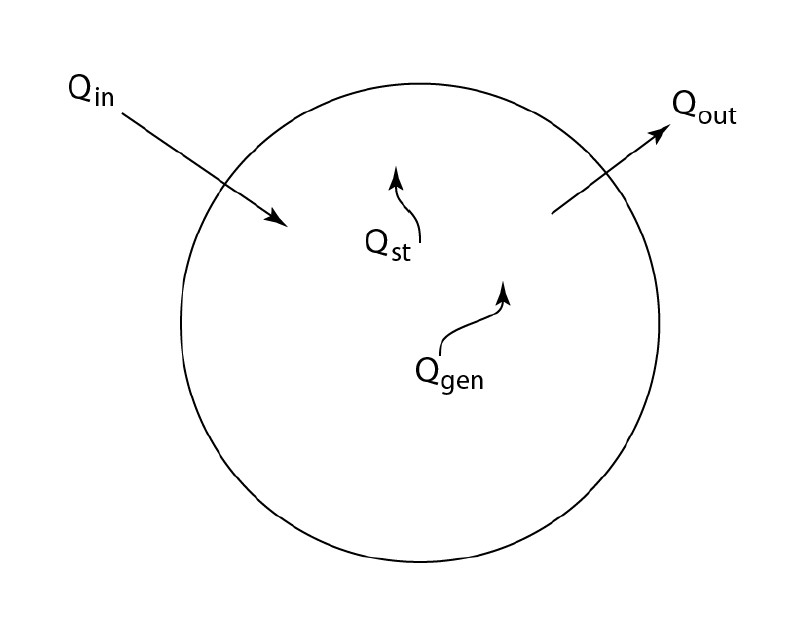A one lump model of heat exchange assuming distributed metabolic heat generation

From Fig. 5, the equation is

$\begin{equation} Q_{in} + Q_{gen} = Q_{out} + Q_{st} \end{equation}$

where

$\begin{equation} Q_{in} = Q_{sol,abs} + Q_{IR,in} \end{equation}$

$\begin{equation} Q_{gen} = q^{'''} \cdot V \end{equation}$

$\begin{equation} Q_{out} = Q_{conv} + Q_{IR,out} + Q_{evap} \end{equation}$

$\begin{equation} Q_{st} = m c_p \frac{dT}{dt}. \end{equation}$

We will assume a parabolic body temperature from core to skin, since the solution to all body geometries with uniformly distributed heat generation is a function of the square of the distance from the center of the body to the surface for each of the cartesian coordinates of heat flow (Bird et al, 2002; Porter, 2016):

$\begin{equation} T_c - T_s = \frac{q^{'''}S^2}{2k} \end{equation}$

$\begin{equation} T_s = T_c - \frac{q^{'''}S^2}{2k}. \end{equation}$

We will also assume that the capacity to generate heat is independent of body temperature, although that is not a necessary prerequisite, it just simplifies the form of the solution. We will assume that during the transient, the environmental conditions do not change in time, i.e. $$Q_{in}$$ and $$Q_{gen}$$ are constant. We will also assume that these two terms are independent of body temperature. The two terms on the right side of the system equation, $$Q_{out}$$ and $$Q_{st}$$, are a function of body temperature. For convenience, we can reverse the order of the terms in the equation, define the temperature dependent terms, solve the equation by integration, then explicitly define the terms that affect the transient.

$\begin{equation} Q_{st} + Q_{out} = Q_{in} + Q_{gen} \end{equation}$

or

$\begin{equation} Q_{st} + Q_{conv} + Q_{IR,out} + Q_{evap} = Q_{sol,abs} + Q_{gen} + Q_{IR,in} \end{equation}$

Here we assume negligible evaporative water loss and delete this nonlinear term, which greatly simplifies the solution.

The long-wavelength infrared heat exchange can be expressed as a net term $$Q_{rad,net} = Q_{IR,in} - Q_{IR,out} = h_{rad} A (T_s - T_{rad})$$ where

$\begin{equation} h_{rad} = 4 \sigma T_{ave}^3, \end{equation}$

is the radiant heat transfer coefficient, as approximated by a Taylor Series expansion where $$T_{ave} = \frac{T_s + T_{rad}}{2}$$, with $$T$$ in Kelvin. This Taylor Series expansion closely approximates the exact Stefan-Boltzmann equation. There is only a 1.1% error when the temperature difference between $$T_s$$ and $$T_{rad}$$ is 60 °C.

Given those assumptions, the equation to solve is now

$\begin{equation} mc_p \frac{dTc}{dt} + h_{conv} A(T_c - \frac{q^{'''}S^2}{2k} - T_a) + h_{rad} A(T_c - \frac{q^{'''}S^2}{2k} - T_{rad}) = Q_{sol,abs} + Q_{gen}. \end{equation}$

Putting all the constants on the right hand side of the equal sign we have

$\begin{equation} mc_p \frac{dT_c}{dt} + (h_{conv} A + h_{rad} A)T_c = Q_{sol,abs} + Q_{gen} + h_{conv} A \frac{q^{'''}S^2}{2k} + h_{rad} A \frac{q^{'''}S^2}{2k} + h_{conv} A T_a + h_{rad} A T_{rad} \end{equation}$

or

$\begin{equation} \frac{dT_c}{dt} + \frac{A(h_{conv} + h_{rad}) }{mc_p}T_c = \frac{Q_{sol,abs} + Q_{gen} + A [\frac{h_{conv} q^{'''}S^2}{2k} + \frac{h_{rad} q^{'''}S^2}{2k} + h_{conv} T_a + h_{rad} T_{rad}]}{mc_p}. \end{equation}$

The groups of variables are all assumed constant so we have an equation of the form

$\begin{equation} \frac{dT_c}{dt} + \theta T = j. \end{equation}$

We solve the ordinary differential equation the same way as before by noting that the solution is the sum of the transient (complimentary) part and the steady state (particular) part solutions, i.e. $$y = y_c + y_p$$. Thus, to solve the transient part we set $$j = 0$$.

$\begin{equation} \frac{dT_c}{dt} + \theta T_c = 0. \end{equation}$

Rearranging

$\begin{equation} \frac{dT_c}{T_c} = -\theta dt. \end{equation}$

Integrating

$\begin{equation} lnT_c = -\theta t + C_1. \end{equation}$

Taking antilogs

$\begin{equation} y_c = T_c = C_1 e^{-\theta t}. \end{equation}$

We do not solve for $$C_1$$ until we have the steady state (particular) solution, which we get by setting $$\frac{dT_c}{dt}$$ to zero

$\begin{equation} 0 + \theta T_c = j \end{equation}$

or

$\begin{equation} y_p = T_c = \frac{j}{\theta}. \end{equation}$

Since $$y = y_c + y_p$$

$\begin{equation} T_c = C_1 e^{-\theta t} + \frac{j}{\theta}. \end{equation}$

Now we solve for the integration constant, $$C_1$$. We use an initial condition at $$t = 0$$, $$T = T_{c,i}$$

$\begin{equation} T_{c,i} = C_1 \cdot (1) + \frac{j}{\theta}. \end{equation}$

Solving for $$C_1$$ and inserting it in the general solution we have

$\begin{equation} T_c = (T_{c,i} - \frac{j}{\theta})e^{-\theta t} + \frac{j}{\theta}. \end{equation}$

The term $$\frac{j}{\theta}$$ is the steady state solution for the final body temperature, $$T_{c,f}$$.

$\begin{equation} T_c = (T_{c,i} - T_{c,f})e^{-\theta t} + T_{c,f}. \end{equation}$

## 7. Derivation of the two-lump solution

When the Biot number is >0.1 there may be enough of a radial dimension that it might seem more reasonable to approximate the animal as having a central core and a peripheral area, each with a different temperature. Figure 2 still applies as the system diagram. Without the approximation of uniform temperature, we have three unkown temperatures, core, $$T_c$$, middle of the skin, $$T_{sk}$$, and outer surface, $$T_s$$. With three unknowns, we will need three simultaneous equations to solve for them. These equations come from energy balances on the three temperature nodes.

At $$T_c$$:

$\begin{equation} Q_{met} - Q_{evap} - \frac{T_c - T_{sk}}{R_b} = C_c \frac{dT_c}{dt}. \end{equation}$

At $$T_{sk}$$

$\begin{equation} \frac{T_c - T_{sk}}{R_b} - \frac{T_{sk} - T_s}{R_{sk} / 2} = C_s \frac{dT_{sk}}{dt}. \end{equation}$

At $$T_o$$

$\begin{equation} \frac{T_{sk} - T_s}{R_{sk} / 2} + Q_{sol} = \frac{T_s - T_{rad}}{R_{rad}} + \frac{T_s - T_a}{R_{conv}}. \end{equation}$

We have two differential equations and a third that can be solved for $$T_s$$. The general tactic here will be to set up the equations in the general form of equation 13 and solve them simultaneously for the particular integral first, then the complimentary function, then finally evaluate the integration constants.

Equation 63 can be rearranged by first segregating $$T_s$$ in fractional form on the left, then factoring it from the fractions to obtain

$\begin{equation} T_s = \frac{Q_{sol} + T_{rad} / R_{rad} + T_{air} / R_{conv} + 2 T_{sk} / R_{sk}}{1/R_{rad} + 1/R_{conv} + 2/R_{sk}}. \end{equation}$

These equations can then be used in conjunction with an ODE solver to compute the transient heat budget under constant or time-varing environmental conditions. The remainder of this document develops an analytical solution however it still includes the term $$R_{rad}$$ which itself has the term $$T_s$$ in it.

Substituting 64 into 62 we have

$\begin{equation} \frac{T_c - T_{sk}}{R_b} - \frac{T_{sk}}{R_{sk}/2} + \frac{Q_{sol} + T_{rad} / R_{rad} + T_{air} / R_{conv} + 2 T_{sk} / R_{sk}}{R_{sk}/2(1/R_{rad} + 1/R_{conv} + 2/R_{sk})} = C_{sk} \frac{dT_{sk}}{dt}. \end{equation}$

Since we have to integrate 65, we will rearrange to get the general form $$\frac{dy}{dt} + ky$$ = forcing function.

Thus, 65 becomes, after rearrangement

$\begin{equation} \frac{dT_{sk}}{dt} = AT_{sk} + BT_c + D \end{equation}$

where

$\begin{equation} A = \frac{1}{C_{sk}}[\frac{1}{R_b} + \frac{2}{R_{sk}} - \frac{2/R_{sk}}{R_{sk}/2 R_{rad} + R_{sk} / 2 R_{conv} + 1}] \end{equation}$

and

$\begin{equation} B = \frac{1}{R_b C_{sk}} \end{equation}$

and

$\begin{equation} D = \frac{Q_{sol} + T_{rad} / R_{rad} + T_{air}/R_{conv}}{(R_{sk} / 2 R_{rad} + R_{sk} / 2 R_{conv} + 1)C_{sk}}. \end{equation}$

Rearranging equation 61 to put in similar form as 66 we have

$\begin{equation} \frac{dT_c}{dt} = [\frac{1}{C_c R_b}]T_{sk} - [\frac{1}{C_c R_b}]T_c + \frac{Q_{met} - Q_{evap}}{C_c} \end{equation}$

or

$\begin{equation} \frac{dT_c}{dt} = FT_{sk} - FT_c + H. \end{equation}$

### The particular integral

Now we can solve equations 66 and 71 for the complimentary (transient) function and the particular (steady state) integral such that $$y = y_c + y_p$$. The particular integral has no change of temperature with time, so from 66 and 71 we have

$\begin{equation} FT_{sk} - FT_c = -H \end{equation}$

and

$\begin{equation} -AT_{sk} + BT_c = - D. \end{equation}$

These equations have the form $$a_1 x + b_1 x = k_1$$ and $$a_2 x + b_2 y = k_2$$, respectively. These equations can be solved simultaneously. A convenient way to do this is to use determinants from linear algebra, where

$\begin{equation} x = \frac{k_1 b_2 - k_2 b_1}{a_1 b_2 - a_2 b_2} \end{equation}$

and

$\begin{equation} y = \frac{a_1 k_2 - a_2 k_1}{a_1 b_2 - a_2 b_1}. \end{equation}$

If we let $$x = T_{sk}^P$$ and $$y = T_{c}^P$$ then

$\begin{equation} T_{sk}^P = \frac{-HB-DF}{FB-AF} \end{equation}$

and

$\begin{equation} T_{c}^P = \frac{-FD-AH}{FB-AF}. \end{equation}$

### The complementary function

The complementary function has the general form $$\frac{dy}{dt} + ky = 0$$. Thus, equations 66 and 71 are

$\begin{equation} \frac{dT_{sk}}{dt} + AT_{sk} = BT_c \end{equation}$

and

$\begin{equation} \frac{dT_c}{dt} + FT_c = FT_{sk}. \end{equation}$

If we use a $$P$$ operator again, we again have two simultaneous algebraic equations to solve,

$\begin{equation} T_{sk}(P+A) = BT_c \end{equation}$

and

$\begin{equation} T_c(P+F) = FT_{sk} \end{equation}$

We can substitute the value of $$T_{sk}$$ from equation 80 into 81 to get an expression for $$T_c$$

$\begin{equation} T_c(P+F) = \frac{FBT_c}{P+A} \end{equation}$

or

$\begin{equation} T_c[P^2 + P(F+A) + FA - FB] = 0. \end{equation}$

Equation 83 has a trivial solution, $$T_c = 0$$, or

$\begin{equation} P^2 + P(F+A) + F(A - B) = 0. \end{equation}$

This has the familiar form of a quadratic equation, $$ax^2 + bx + c = 0$$. Its roots are

$x = \frac{-b \pm \sqrt{b^2 - 4ac}}{2a}$

or

$\begin{equation} P = \frac{-(F + A) \pm \sqrt{(F+A)^2 - 4FA + 4FB}}{2} = P_1P_2. \end{equation}$

There are three possibilities for the values of $$P$$ depending on whether the quantity under the radical is positive, zero or negative. If the quantity is positive, as in our case, the solution is $$y = C_1 e^{P_1t} + C_2 e^{P_2t}$$ so, since $$y = y_c + y_p$$ we have

$\begin{equation} T_c = C_1 e^{P_1t} + C_2 e^{P_2t} - \frac{FD + AH}{FB - AF}. \end{equation}$

We still need to determine the values of the integration constants $$C_1$$ and $$C_2$$. To get them, we will need two equations. Intitial conditions at $$t = 0$$, where $$T_c = T_{c,0}$$, is an easy time dependent condition. Substituting into equation 86 we have

$\begin{equation} T_{c,0} = C_1 + C_2 - \frac{FD + AH}{FB - AF}. \end{equation}$

We can use a ‘trick’ to get the second equation needed to evaluate the two integration constants. We can take the derivative of 86

$\begin{equation} \frac{dT_c}{dt} = C_1 P_1 e^{P_1t} + C_2 P_2 e^{P_2t}. \end{equation}$

We can now replace the deriviative and then the definition of $$T_c$$ into equation 87 using equations 71 and 86. This gives

$\begin{equation} C_1 P_1 e^{P_1t} + C_2 P_2 e^{P_2t} = F T_{sk} - F[C_1 e^{P_1t} + C_2 e^{P_2t} - \frac{FD + AH}{FB - AF}] + H. \end{equation}$

At $$t = 0$$,

$\begin{equation} C_1 P_1 + C_2 P_2 = F T_{s,0} - F[C_1 + C_2 - \frac{FD + AH}{FB - AF}] + H. \end{equation}$

We now have two equations, 88 and 90, that we can use to solve for the two integration constants, $$C_1$$ and $$C_2$$. Solving for the integration constants and defining a new constant for the right hand side for algebraic simplicity we have

$\begin{equation} C_1 + C_2 = T_{c,0} + \frac{A+D}{B-A} = \alpha \end{equation}$

and

$\begin{equation} C_1(P_1 + F) + C_2(P_2 + F) = FT_{sk,0} + \frac{HB-FD}{B-A} = \beta. \end{equation}$

We can solve these using equations 74 and 75 to solve for $$C_1$$ and $$C_2$$

$\begin{equation} C_1 = \frac{\alpha (P_2 + F) - \beta}{(P_2 + F) - (P_1 + F)} = \frac{\alpha(P_2 + F) - \beta}{P_2 - P_1} \end{equation}$

and

$\begin{equation} C_2 = \frac{\beta - \alpha(P_1 + F)}{P_2 - P_1}. \end{equation}$

Substituting 93 and 94 into 86 we have

$\begin{equation} T_c = [\frac{\alpha(P_2 + F) - \beta}{P_2 - P_1}]e^{P_1t} + [\frac{\beta - \alpha(P_1 + F)}{P_2 - P_1}]e^{P_2t} - \frac{FD + AH}{F(B-A)} \end{equation}$

or

$\begin{eqnarray} \begin{split} \begin{gathered} T_c = [\frac{[T_{c,0} + \frac{A+D}{B-A}](P_2 + F) - [F T_{sk,0} + \frac{HB-FD}{B-A}]}{P_2-P_1}]e^{P_1t} \\ + [\frac{[F T_{s,0} + \frac{HB-FD}{B-A}] - [T_{c,0} + \frac{A+D}{B-A}](P_1 + F)}{P_2-P_1}]e^{P_2t} \\ - \frac{FD + AH}{F(B-A)}. \end{gathered} \end{split} \end{eqnarray}$